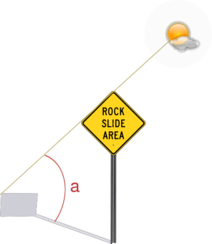homechevron_rightStudychevron_rightAstronomy

# Shadow length

Gives a vertical object shadow length for given coordinates and time. Also calculates shadow growth during whole day.Sun altitude and shadow

This simple online calculator gives a vertical object shadow length for specified day and geographic coordinate. The calculator uses Sun position algorithm to calculate sun altitude.
Then it uses this formula to calculate shadow length:

,

where

h - object height,
a - angle between Sun and horizon.

The calcultor gives zero result if there is no shadow. As you know, this may happen at night time :).### Shadow length

::

°

°

Digits after the decimal point: 2
Shadow length

You may also calculate the shadow growth during the whole day using the calculator below:### Daily shadow growth

°

°

Digits after the decimal point: 2
Shadow length

## Similar calculators

636 calculators in total.